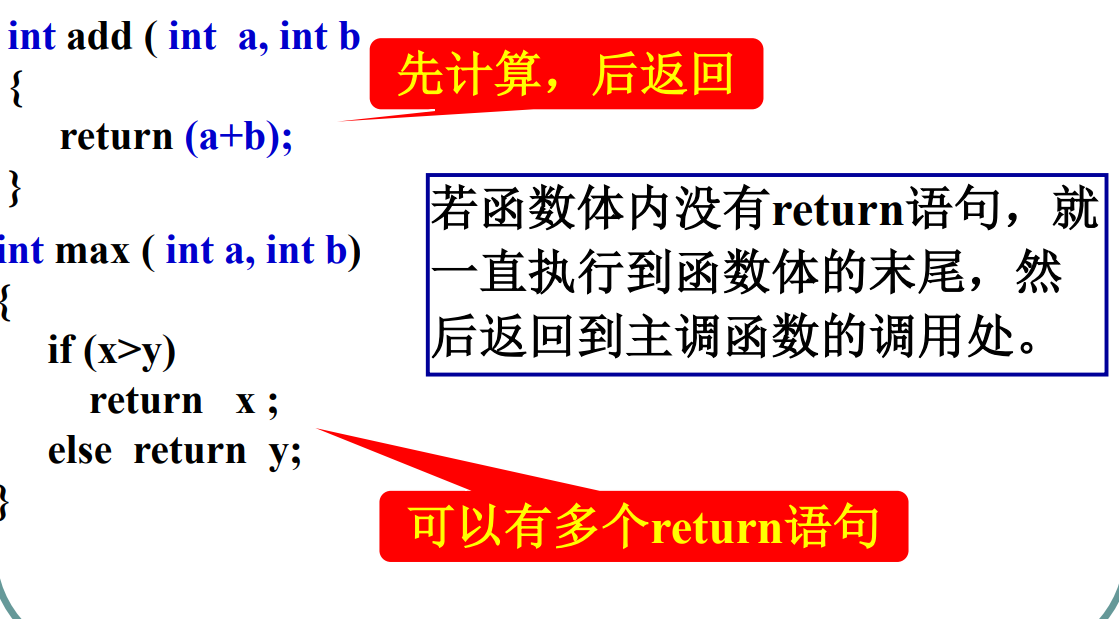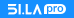# 掌握PHP关键字，让函数执行结果完美返回

a)、 // 返回一个表达式结果

b)、(expr) // 函数表达式

c)、 // 直接返回，或返回空值1、如果执行了，则 语句后面的内容将不会被执行了；

```function add(\$a,\$b){
return \$a+\$b;
return \$a*\$b;
}
echo \$c;```

2、 可以是函数返回值，也可以返回一个空值，这要看具体用法，例如：```function test(\$a){
if(\$a>10){
return "a>10";
}else{
return "a<10";
}
\$b=45;
\$c=\$b-\$a;
echo \$c;
}```

```  \$b=45;
\$c=\$b-\$a;
echo \$c;```

3、关于的调用。a.php如下：

b.php如下：a.php如下：

b.php如下：4、的一个重要作用：返回值

```function test(){
\$a=array(1,2);
return \$a;
}
\$b=test();
print_r(\$b);```

```function test(){
\$a=array(1,2);
}
\$b=test();
print_r(\$b);```

(云端网 )

www.ydw.org - 云端网

该栏目下无二级栏目777777  666666